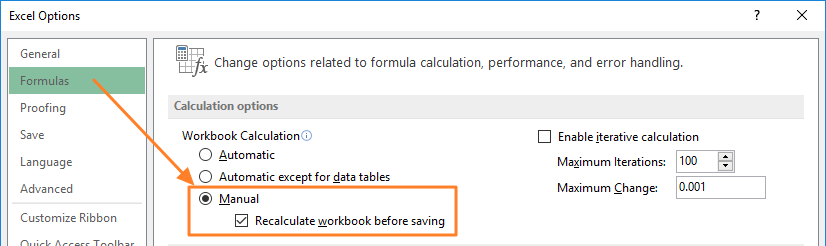As the number of formulas and data in your workbook increase, so will calculation times. If you're at a point where you need to wait a while after every action, you should probably consider switching to manual calculations. In this article, we're going to show you how to enable manual calculations, if Excel is running slow.

Calculation options in Excel can be set in 2 ways:

1. FORMULAS > Calculation Options2. FILE > Options > Formulas > Calculation optionsThe difference between the 2 methods is that Recalculate workbook before saving property in the Options window will only show in the second one. This property forces calculating the entire workbook before any save action.

Please note that the calculation mode works the same for all open workbooks regardless of how they are saved. In other words, if you open a workbook with calculations set to Automatic after opening a workbook with manual calculations, the second workbook will run with manual calculations.

After setting the calculation mode to Manual, you can use any of the methods below to run calculations:

 Action Shortcut Calculate all worksheets in all open workbooks F9 Calculate the active worksheet Shift+F9 Calculate all worksheets in all open workbooks, regardless of whether they have changed since the last calculation Ctrl+Alt+F9 Check dependent formulas, and then calculate all cells in all open workbooks, including cells not marked as needing to be calculated Ctrl+Alt+Shift+F9

Alternatively, you can use the Calculate Now and Calculate Sheet buttons in the ribbon. These do the same thing as pressing F9 and Shift+F9 respectively.